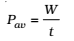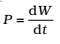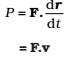Power is defined as the time rate at which work is done or energy is transferred.

The average power of a force is defined as the ratio of the work, W, to the total time t takenThe instantaneous power is defined as the limiting value of the average power as time interval approaches zero,The work dW done by a force F for a displacement dr is dW = F.dr. The instantaneous power can also be expressed aswhere v is the instantaneous velocity when the force is F.

Power, like work and energy, is a scalar quantity. Its dimensions are [M L2 T-3]. In the SI, its unit is called a watt (W). The watt is 1 J s-1.
The unit of power is named after James Watt, one of the innovators of the steam engine in the eighteenth century.
There is another unit of power, namely the horse-power (hp)
1 hp = 746 W